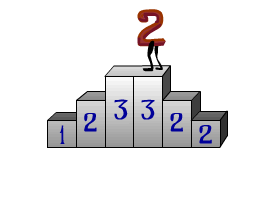Problems

# Degree of symmetryFor a natural number let's define the degree of symmetry as an amount of pairs of its decimal digits, whose digits are equal and stand symmetrically with respect to the center of the number's decimal notation. If some digit stands in the center of the decimal notation, then it must be accepted too, as in pair with itself. Find the degree of symmetry of a given number n.

#### Input

The natural number n (n < 2 ·109).

#### Output

Print the degree of symmetry of n.

Time limit 1 second
Memory limit 64 MiB
Input example #1
123322

Output example #1
2